Kinematic Self-Replicating Machines

Robert A. Freitas Jr., Ralph C. Merkle, Kinematic Self-Replicating Machines, Landes Bioscience, Georgetown, TX, 2004.

5.1.9.G Replicator Energetics

G1. Process Energy Autonomy.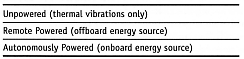G2. Process Energy Redundancy. This refers to the number of onboard energy conversion mechanisms – as for example the number of independent glucose engines inside each respirocyte . Replicator reliability is enhanced by providing greater redundancy of onboard energy sources.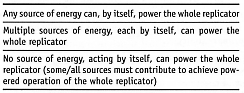G3. Process Energy Centralization. How many sources of energy are there?G4. Number of Onboard Process Energy Types. How many different types of energy are employed? A replicator which is capable of replicating using multiple kinds of energy (e.g., chemical, acoustic, photonic, etc.) is more versatile and can operate in a greater range of environments and conditions, and is probably also more complex, hence is less likely to remain “inherently safe” (Section 5.11).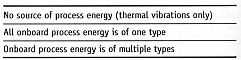G5. Process Energy Types. A matrix of power conversion processes that might be employed by replicators is readily defined (Nanomedicine , Section 6.3 and Table 6.2).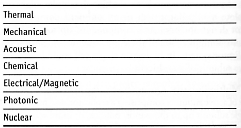G6. Process Energy Storage. Can energy be stored onboard the replicator (e.g., in batteries)? If energy can be stored onboard, can those stores be replenished during normal operations?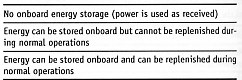G7. Process Energy Efficiency. This factor is intended to be a quantitative measure of the amount of energy consumed in a single replication, possibly scaled to system mass, called the “energy efficiency” or more particularly the “specific energy for replication” (e). For example, the synthesis of one (Mmicrobe = 2.5 x 10-16 kg) E. coli cell from glucose may consume three times the microbe’s weight in glucose (4.2 x 10-9 µmoles) , or Emicrobe = 1.2 x 10-8 J, giving an energy efficiency of emicrobe = Emicrobe / Mmicrobe = 4.8 x 107 J/kg for replication. This is very good for an irreversible process, when compared to the range of e ~ 105 J/kg (for practical nearly-reversible mill-style manufacturing processes; Nanosystems , Section 13.3.7.b) to e ~ 108 J/kg for chemical transformations envisioned for molecular manufacturing (Nanosystems , Section 13.3.7.b; Nanomedicine , Section 6.5.6(B)). By contrast, the first-generation Merkle-Freitas molecular assembler (Section 4.11.3.1) would be very energy inefficient, consuming EMF = 1.49-5.93 x 10-5 J to replicate one MMF = 3.90826 x 10-15 kg device, a much poorer energy efficiency of eMF ~ 0.38-1.5 x 1010 J/kg for a single replication. Note that in biological systems of mass M, replicator metabolic rate B (watts) ~ kmet M3/4 , hence the specific energy for replication scales as erep ~ kmet · trep · M-1/4 (J/kg), where trep is replication time and kmet is a proportionality constant. Since trep ~ M1/4 (Figure 5.6), then erep is independent of mass, at least for biological replicators. The possibility that different scaling laws might apply to molecular assemblers or nanofactories should be investigated further (Sections 5.2 and 5.9.5).

Process energy efficiency can also be scaled to system information content. For example, following a related suggestion by C. Phoenix  we can define an alternative dimensionless measure of energy efficiency (perhaps to be called the “replication informatic energy ratio” or eI) as the ratio of the amount of energy actually used to manufacture a replica to the kT entropic value of the information needed to describe the replicator. The most efficient possible replicator would have a ratio of eI = 1. Taking T = 300 K as the operating temperature, an E. coli requiring Imicrobe = 9.278442 x 106 bits to describe (ignoring genomic redundancy; Table 5.1) has a replication informatic energy ratio of eI = Emicrobe / (kT ln(2) · Imicrobe) = 4.5 x 105. The Merkle-Freitas molecular assembler requires IMF ~ 50.0 x 106 bits to describe (assuming data compression; Table 5.1), yielding a replication informatic energy ratio of only eI = EMF / (kT ln(2) · IMF) = 1.0-4.1 x 108, or 2-3 orders of magnitude less energy efficient than E. coli. Note that “replicators that are inefficient in structure (e.g., [molecular assemblers or nanofactories] using vast blocks of diamondoid) will also be inefficient by this measure, since such non-complex structure will not require many bits to specify.”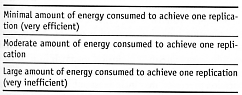G8. Replicative Energy Commitment. What percentage of total processing energy is devoted to replicative activities? In biology, larger replicators generally use a lesser fraction of their total systemic energy for reproduction than do smaller replicators .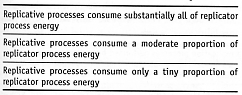Last updated on 1 August 2005